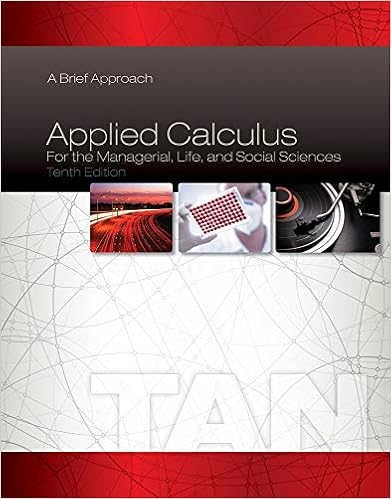# There are two problems with the trade that i have

• Notes
• 152
• 100% (1) 1 out of 1 people found this document helpful

This preview shows page 143 - 146 out of 152 pages.

##### We have textbook solutions for you!
The document you are viewing contains questions related to this textbook.The document you are viewing contains questions related to this textbook.
Chapter 2 / Exercise 5
Applied Calculus for the Managerial, Life, and Social Sciences: A Brief Approach
TanExpert Verified
the additional unit that firm two produces). There are two problems with the trade that I have made. First, I have increased the total cost to firm two, which must now do more of the pollution reduction, and reduced the total cost to firm one, which must now do less of the pollution reduction—I have rewarded the ine cient firm and penalized the e cient firm. Second, to make this trade I must know the marginal cost curves for both firms. This is information that the government is unlikely to
##### We have textbook solutions for you!
The document you are viewing contains questions related to this textbook.The document you are viewing contains questions related to this textbook.
Chapter 2 / Exercise 5
Applied Calculus for the Managerial, Life, and Social Sciences: A Brief Approach
TanExpert Verified
136 CHAPTER 9. EXTERNALITIES Figure 9.6: A Reduction in the Total Cost of Reducing Pollution have. 9.4.2 Transferable Pollution Rights A solution to these problems is transferable pollution rights. Under transfer- able pollution rights, each firm has the right to pollute a fixed amount and firms are allowed to trade their pollution rights with one another. That is, a firm can sell to another firm the right to emit X units of pollution. If a firm makes such a sale, it must increases its pollution reduction by X units since it no longer has the right to emit those X units of pollution. Two firms will make such a transaction if their marginal costs of reducing pollution are di ff erent. To see why, consider a price (per unit of pollution right) that is between the marginal costs of the firms. At this price, the low cost can firm sell a pollution unit for more than it costs it to reduce pollution by one unit. The high cost firm, meanwhile, can pay less for the right to pollute one unit than it is currently paying to reduce its last unit of pollution. Thus, the trade increases both firms’ profits. Consider, for example, figure 9.6. Assume that at R G both firms are using their full allocation of pollution rights. Then it is an mutually beneficial
9.4. POLICY OPTIONS 137 trade for firm two to sell one unit of its pollution rights to firm one. Firm two must therefore increase its pollution reduction by one unit while firm one can decrease its pollution reduction by one unit. This transaction makes both firms better o ff without changing the total amount of pollution reduction. In addition, it does not penalize firm two—and e cient firms in general--since firm two can sell its pollution rights to a less e cient firm and increase its total profit. Under this system, firm two will sell pollution rights to firm one until the marginal cost of reducing pollution is equal for both firms. This is the lowest cost at which the government’s desired pollution reduction can be done. 9.4.3 E ffl uent Fees Another possible solution to the problem of pollution is to charge firms a price P AP for every unit of pollution that they create 1 . This strategy, known as e ffl uent fees, is shown in figure 9.7. Firms will choose to reduce pollution up until the point where the marginal cost of reducing pollution equals the price of a unit of pollution. Why? Because when MC < P it is cheaper to reduce pollution than to pay the e ffl uent fee. When MC > P , on the other hand, it is cheaper to pay the e ffl uent fee.
•••